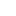• ### Vacuum stability of the two-Higgs-doublet model with CP violation

分类： 数学 >> 数学物理 分类： 物理学 >> 基本粒子与场物理学 提交时间： 2023-01-18宋义生

In this paper, we present how to calculate the bounded from below or the vacuum stability  of scalar potential for a general CP violating two-Higgs-doublet model  by using the concepts of co-positivity and the gauge orbit spaces.  Meanwhile, the semi-positive definiteness is prove for a class of 4th-order 2-dimensional complex tensor.

同行评议状态:待评议

• ### Co-positivity of tensors and Stability conditions of two Higgs potentials

分类： 物理学 >> 基本粒子与场物理学 提交时间： 2022-04-02宋义生

We give how to calculate the vacuum stability  conditions of scalar potential for two Higgs doublet model with explicit CP conservation. Moreover, the analytical sufficient and necessary conditions are obtained. The argument methods are first used to study the tensor with two parameters.

同行评议状态:待评议

• ### Copositivity for a class of fourth order symmetric tensors given by scalar dark matter

分类： 数学 >> 数学物理 提交时间： 2021-06-16宋义生 李旭东

摘要：In this paper, we mainly discuss the copositivity of 4th order symmetric tensor defined by scalar dark matter stable under a $\mathbb{Z}_{3}$ discrete group, and obtain an analytically necessary and sufficient condition of the copositivity of such a class of tensors. Furthermore, this analytic expression may be used to verify the vacuum stability for $\mathbb{Z}_{3}$ scalar dark matter.

同行评议状态:待评议

• ### A necessary and su#14;cient condition of positive definiteness for 4th order symmetric tensors defined in particle physics

分类： 数学 >> 数学物理 提交时间： 2020-11-23宋义生 祁力群

摘要： In this paper, we mainly discuss analytical expressions of positive definiteness for a special 4th order 3-dimensional symmetric tensor defined by the constructed model for a physical phenomenon. Firstly, an analytically necessary and sufficient conditions of 4th order 2-dimensional symmetric tensors are given to test its positive definiteness. Furthermore, by means of such a result, a necessary and sufficient condition of positive definiteness is obtained for a special 4th order 3-dimensional symmetric tensor. Such an analytical conditions can be used for verifying the vacuum stability of general scalar potentials of two real singlet scalar fields and the Higgs boson. The positive semi-definiteness conclusions are presented too.

同行评议状态:待评议

• ### Copositivity for 3rd order symmetric tensors and applications

分类： 数学 >> 数学物理 提交时间： 2019-11-23刘佳蕊 宋义生

摘要： The strict opositivity of 4th order symmetric tensor may apply to detect vacuum stability of general scalar potential. For finding analytical expressions of (strict) opositivity of 4th order symmetric tensor, we may reduce its order to 3rd order to better deal with it. So, it is provided that several analytically sufficient conditions for the copositivity of 3th order 2 dimensional (3 dimensional) symmetric tensors. Subsequently, applying these conclusions to 4th order tensors, the analytically sufficient conditions of copositivity are proved for 4th order 2 dimensional and 3 dimensional symmetric tensors. Finally, we apply these results to present analytical vacuum stability conditions for vacuum stability for $\mathbb{Z}_3$ scalar dark matter.

同行评议状态:待评议

• ### Analytical expressions of copositivity for 4th order symmetric tensors and applications

分类： 数学 >> 控制和优化 提交时间： 2019-08-30宋义生 祁力群

摘要： In particle physics, scalar potentials have to be bounded from below in order for the physics to make sense. The precise expressions of checking lower bound of scalar potentials are essential, which is an analytical expression of checking copositivity and positive definiteness of tensors given by such scalar potentials. Because the tensors given by general scalar potential are 4th order and symmetric, our work mainly focuses on finding precise expressions to test copositivity and positive definiteness of 4th order tensors in this paper. First of all, an analytically sufficient and necessary condition of positive definiteness is provided for 4th order 2 dimensional symmetric tensors. For 4th order 3 dimensional symmetric tensors, we give two analytically sufficient conditions of (strictly) cpositivity by using proof technique of reducing orders or dimensions of such a tensor. Furthermore, an analytically sufficient and necessary condition of copositivity is showed for 4th order 2 dimensional symmetric tensors. We also give several distinctly analytically sufficient conditions of (strict) copositivity for 4th order 2 dimensional symmetric tensors. Finally, we apply these results to check lower bound of scalar potentials, and to present analytical vacuum stability conditions for potentials of two real scalar fields and the Higgs boson.

同行评议状态:待评议

• ### Upper bounds for Z$_1$-eigenvalues of generalized Hilbert tensors

分类： 数学 >> 数学（综合） 提交时间： 2017-12-12孟娟 宋义生

摘要：In this paper, we introduce the concept of Z$_1$-eigenvalue to infinite dimensional generalized Hilbert tensors (hypermatrix) $\mathcal{H}_\lambda^{\infty}=(\mathcal{H}_{i_{1}i_{2}\cdots i_{m}})$, $$\mathcal{H}_{i_{1}i_{2}\cdots i_{m}}=\frac{1}{i_{1}+i_{2}+\cdots i_{m}+\lambda},\ \lambda\in \mathbb{R}\setminus\mathbb{Z}^-;\ i_{1},i_{2},\cdots,i_{m}=0,1,2,\cdots,n,\cdots,$$ and proved that its $Z_1$-spectral radius is not larger than $\pi$ for $\lambda>\frac{1}{2}$, and is at most $\frac{\pi}{\sin{\lambda\pi}}$ for $\frac{1}{2}\geq \lambda>0$. Besides, the upper bound of $Z_1$-spectral radius of an $m$th-order $n$-dimensional generalized Hilbert tensor $\mathcal{H}_\lambda^n$ is obtained also, and such a bound only depends on $n$ and $\lambda$.

同行评议状态:待评议